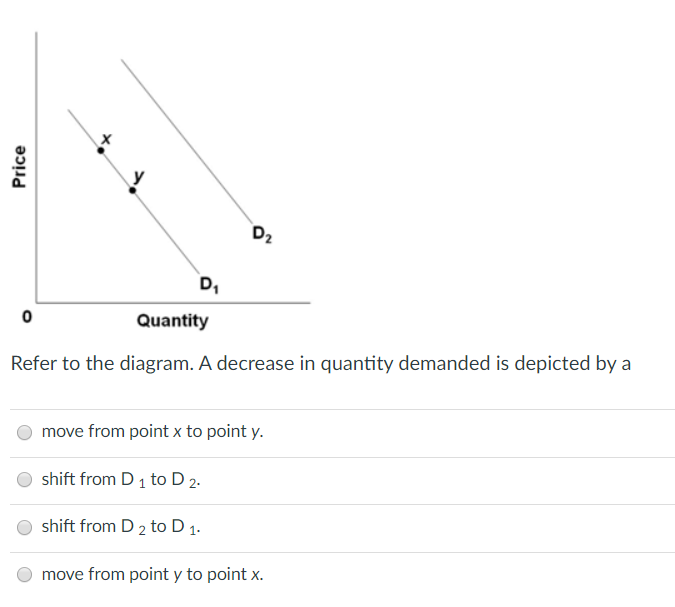# Refer to the diagram. a decrease in quantity demanded is depicted by a

Refer to the diagram. A decrease in quantity demanded is
depicted by aх Price D2 D 0 Quantity Refer to the diagram. A decrease in quantity demanded is depicted by a move from point x to point y. shift from D 1 to D 2. shift from D2 to D 1. move from point y to point x.

If demand is decreases then demand curve shifts to the left.
So demand is decreases then is depicted by a shift from D2 to
D1.
but in the question quantity demand is decreases then move from
point y to point x.
so option D is the correct statement